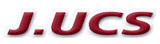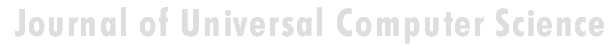Search
 Submission Procedure share: |available in:   PDF (149 kB) PS (47 kB)

get:
 Similar Docs BibTeX Write a comment
get:

DOI:   10.3217/jucs-001-12-0811

Exploiting Parallelism in Constraint Satisfaction for Qualitative Simulation

Marco Platzner
(Graz University of Technology, Austria
marco@iti.tu-graz.ac.at)

Bernhard Rinner
(Graz University of Technology, Austria
rinner@iti.tu-graz.ac.at)

Reinhold Weiss
(Graz University of Technology, Austria
rweiss@iti.tu-graz.ac.at)

Abstract: Constraint satisfaction is very common in many artificial intelligence applications. This paper presents results from parallelizing constraint satisfaction in a special application --- the algorithm for qualitative simulation QSim [Kuipers 94]. A parallel-agent based strategy (PAB) is used to solve the constraint satisfaction problem (CSP). Two essential steps of PAB are studied in more detail to achieve a good performance of the parallel algorithm. Partitioning heuristics to generate independent parts of the overall search space are investigated. Sequential CSP algorithms are compared in order to reveal the most efficient one for QSim. The evaluation of these heuristics and algorithms is based on runtime measurements using CSPs traced from QSim. These runtimes allow a best- and worst-case estimation of the expected speedup of the parallel algorithms. The comparison of sequential CSP algorithms leads to following strategy for solving partitioned problems. Less complex problems are solved with simple backtracking, and more complex models are solved with graph-directed backjumping (GBJ).

Key Words: Parallel constraint satisfaction, QSim, distributed AI

Category: I.2.11, F.2.2, C.3

1 Introduction

Constraint satisfaction is very common in artificial intelligence applications, and it is also a basic operation in qualitative simulation. Constraint satisfaction problems (CSP) are often solved by backtracking algorithms, which find solutions with depth-first search. Many sequential and parallel algorithms have been developed to solve CSPs more efficiently. This paper presents results of our work in parallelizing and distributing constraint satisfaction for the special application QSim.

QSim, the widely-used algorithm for qualitative simulation, has been developed by Kuipers [Kuipers 94]. Qualitative simulation is a new and challenging simulation paradigm. Major areas of qualitative simulation applications are design, monitoring, and fault-diagnosis. A drawback of current QSim implementations is poor execution speed. In our research project [Platzner, Rinner, Weiss 95] a special-purpose computer architecture for QSim is developed to improve the performance. Better performance is achieved by SW/HW-migration of frequently

Page 811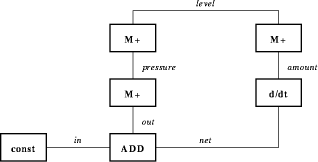Figure 1: Constraint graph of the QSim bathtub model. Constraints are represented by the nodes of the graph. The constraint arity ranges from 1 to 3. Edges between nodes correspond to shared variables. Sets of valid tuples are attached to all constraints after constraint-filtering.

used primitive functions, and mapping QSim kernel functions onto a multiprocessor system. The overall application-specific computer architecture consists of digital signal processors TMS320C40 which are equipped with specialized FPGA-based coprocessors for executing the primitive functions.

2 Constraint Satisfaction in QSim

A constraint satisfaction problem can be informally described as follows: Given a set of n variables each with an associated domain, and given a set of constraints each involving a subset of the variables, find an n-tuple such that this n-tuple is an instantiation of the n variables satisfying all constraints. A more formal description can be found in [Dechter 92].

Constraint satisfaction is a basic operation in the qualitative simulator QSim. It is used to determine all possible successors of a given qualitative state --- i.e. calculating all solutions of a CSP, specified by a constraint network (variables and constraints) and possible values of all variables (domains). In QSim CSPs are represented dual to the representation in [Mackworth 77]. The nodes of the constraint graph correspond to constraints, and the edges between the nodes correspond to variables. A constraint graph of a QSim example is shown in [Figure 1]. This dual representation is used because the arity of QSim constraints is not limited by 2.

Since solving CSPs is NP-complete, preprocessing or filtering steps before backtracking can eliminate large parts of the overall search space [Mohr, Henderson 86]. These techniques are node, arc, and path consistency and are widely applied in constraint satisfaction. In QSim node consistency is achieved by the constraint-filter. For each QSim constraint all possible tuples of the attached variables are checked against the constraint conditions. Tuples violating these conditions are discarded. Arc consistency is achieved by the Waltz-filter, which eliminates inconsistencies between adjacent constraints. Each tuple associated with constraint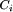is discarded unless the same value of the shared variable is assigned in at least one tuple of each adjacent constraints. Path consistency is not currently used in QSim. The final backtracking step generates all valid assignments of

Page 812

the remaining tuples and thus all solutions of the CSP. A simple backtracking algorithm is used for this depth-first search. Increased performance is achieved by interleaving node and arc consistency algorithms and by a heuristic ordering of the constraints for the backtracking step.

There are many techniques exploiting the parallelism of these filtering steps [Conrad, Agrawal, Bahler 92][Cooper, Swain 92]. We also parallelize the node and arc consistency algorithm of QSim in our research project, but in this paper we present only the results in parallelizing and distributing the backtracking algorithm [Riedl 95].

CSPs in QSim have special characteristics which are different from many other CSPs. These characteristics have to be considered in selecting appropriate algorithms and parallelizing techniques as presented in the next section.

number of solutions
QSim needs all solutions of the CSP for further processing. Searching cannot be finished after finding one solution. All parts of the search space have to be checked. This is different from many other applications, where just one solution is required. Efficiency considerations for these applications can be found in [Rao, Kumar 93].
variable domain
Pure qualitative simulation uses discrete variables and the number of values is limited in most cases by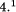arity of the constraints
QSim describes the simulation model with different constraints. The arity of the most important constraints ranges from 1 to 3.
structure of the CSP
In qualitative simulation CSPs with the same structure --- CSPs with the same constraints and variables but different domains --- often have to be solved successively. The description of such CSPs can be simplified, a representation of the domain of the variables is sufficient.Calculating the initial states from an incomplete state description can lead to more than 4 values of individual variables.

3 Parallel Constraint Satisfaction

3.1 Existing Algorithms

Many parallel algorithms for constraint satisfaction are known in literature. Luo, Hendry, and Buchanan [Luo, Hendry, Buchanan 94] have classified the most common algorithms as distributed-agent-based (DAB), parallel-agent-based (PAB), and functional-agent-based (FAB). Different strategies involve different control structures, problem spaces, and communication methods. The FAB strategy can be excluded from further considerations, because it requires shared memory architectures. Important features of the remaining strategies can be summarized as follows.

DAB
In the DAB strategy, the problem is distributed based on the variables. Each agent controls one or more variables and their domains. The search space is shared among the agents and the agents have to communicate because of the constraints between distributed variables.
PAB
In the PAB strategy, the problem is distributed based on the domains of the variables. Each agent solves a part of the complete search space, which is independent from each other, because each search space involves all

Page 813

variables. Therefore, all agents solve a unique CSP and no communication between agents is required.

Our application-specific computer architecture has to fulfill several requirements, which obviously influence the parallelization of the constraint satisfaction algorithm. The following requirements are of special interest.

scalability
Our special-purpose computer architecture consists of several independent processors, each equipped with its own local memory. The number of processing elements is moderate but variable. Thus, the parallel constraint satisfaction algorithm should be scalable.
model independence
The parallel algorithm should be applyable to all QSim models --- i.e. the application of the algorithm should not depend on the structure of the model (CSP graph).

There are several reasons for choosing a PAB strategy for our application. First, it is an excellent strategy for finding all solutions of a given CSP and it is an inherent scalable algorithm. Second, the PAB strategy can be applied to problems with arbitrary structure. Finally, the independent search spaces can be solved with any sequential (and optimized) algorithm. A detailed comparison between DAB and PAB strategies can be found in [Luo, Hendry, Buchanan 94].

In the next two subsections, we consider the essential steps of PAB in more detail --- we investigate methods to achieve a good partitioning of the complete search space, and compare sequential algorithms to use the best one for QSim- models and their partitioned subproblems.

3.2 Generating Independent Subproblems

3.2.1 Evaluation and Speedup Estimation

Partitioning of the complete search space is essential for an efficient parallel algorithm. The partitioning methods are evaluated using runtimes of the subproblems. The most interesting runtimes are the overall runtime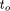, which is the sum of the runtimes of all subproblems, the maximum runtime of all subproblems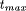, and the sequential runtime of the unpartitioned problem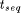. Due to redundancies in the independent subproblems the overall runtimecan be longer than. An efficient partitioning method keeps the overall runtime small. Ifgets smaller thana superlinear speedup is expected. The subproblem with the longest runtime restricts the maximum speedup. Thus, a balanced partitioning, where all subproblems have nearly the same runtime, should be achieved.

Using these runtimes (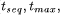and), it is possible to estimate the speedup of the parallel algorithm. Communication times are not considered in this estimation, and simple task attraction is assumed to schedule tasks to free processors. We determine the limits of the speedup by worst- and best-case estimation. First of all, the speedup is defined as S(n) =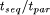, where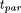denotes the runtime using n processors.

The worst-case condition is satisfied if the longest task is scheduled last and all other tasks are equally distributed among the processors. The worst case runtime of the parallel algorithm can be given as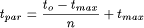Page 814

For best case estimation we have to consider two cases. If the number of processors is greater than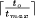the parallel runtime is limited by, otherwise all tasks are equally divided among the processors. More formally, the best case parallel runtime can be estimated as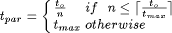3.2.2 Partitioning Methods

Two partitioning methods are investigated --- constraint-based partitioning and variable-based partitioning.

constraint-based partitioning (CBP)
This partitioning is based on the tuple sets of the constraints. The tuple set of an individual constraint is divided into two or more disjunct subsets. A subproblem is defined by one subset and the tuple sets of the remaining constraints. Thus, two or more subproblems are generated. To achieve more subproblems than elements of one tuple set partitioning is extended recursively.

Two variants of this method are studied. CBP-ALL divides the tuple set of the constraint in as many subsets as elements in the tuple set. CBP-ALL tries to generate tuple sets with just one tuple. All variables of such constraints can be instantiated before backtracking starts. CBP-HALF divides the tuple set into two parts. Hence, more tuple sets can be divided and the overall number of tuples in the subproblems is a little smaller.

variable-based partitioning (VBP)
The tuple sets of adjacent constraints are not independent from each other. The tuple sets depend on the domain of the shared variables. This dependency is exploited by the VBP method. The domain of the variable is divided into two or more subdomains. This induces a partitioning of the tuple sets of all attached constraints. In an individual subset there are only the same values of the shared variable as in the corresponding subdomain. Combinations of subsets with different values of the shared variable are inconsistent and can be discarded. Hence, as many subproblems as subdomains are generated. To generate more subproblems than the ordinality of one domain, partitioning is extended to other variables. Four variants of VBP can be classified by the sequence of variables which domains are partitioned. VBP-INST uses the same order as the sequential algorithm. Variables which are shared by many constraints are partitioned first by VBP-CON. VBP-DOM divides the largest domains first. Finally, VBP-TUP takes variables with the largest number of attached tuples first --- i.e. the order of the variables is given by the number of tuples of the attached constraints.

3.3 Solving the Subproblems

With the parallel CSP strategy PAB the individual subproblems can be solved with any sequential algorithm. QSim uses a simple backtracking algorithm, extended by a constraint ordering scheme, for this task. There are many extensions and improvements of simple backtracking known in literature. [Prosser 93]

Page 815

presents an overview of possible improvements, other enhancement schemes are also presented in [Dechter 90]. Most of these improvements were evaluated with standard CSP benchmarks (ZEBRA problem, N-queens, randomly generated CSPs, etc.).

QSim CSPs have different characteristics than those benchmark CSPs. Obviously we are interested in fast algorithms for QSim CSPs. Therefore, we evaluate improved backtracking algorithms with CSPs traced from QSim. These algorithms are: FC (forward checker), CBJ (conflict-directed backjumping), and GBJ (graph-directed backjumping). A simple backtracking algorithm (BT) is also executed as a reference. The implementation of these algorithms is based on [Kondrak 94] and the CSP-library of [Beck 94].

4 Experimental Results

4.1 QSim CSPs

To obtain realistic results from our measurements, three different QSim models have been simulated and the generated CSPs have been traced. Two simulation models were chosen from the QSim-package. The Starling model (STLG) has 17 variables and 18 constraints, and the Heart model (HEART) consists of 28 variables and 21 constraints. The Reaction-Control-System (RCS) [Kay 92], which is not included in the QSim-package, is the most complex model we have traced. It consists of 45 variables and 48 constraints.

8 to 16 CSPs with different complexity --- different cardinality of the variables' domains --- were chosen from the big number of CSPs generated during qsim runs. The CSPs were executed on a digital signal processor TMS320C40. The runtimes of all backtracking algorithms were measured with the internal hardware timer of this processor.

4.2 Partitioning Methods

The most interesting runtimes for evaluating the two partitioning methods (CBP and VBP) and its variants are presented in [Table 1] and [Table 2]. Only the runtime of the backtracking algorithm for solving the subproblems is shown in these tables. The overall runtime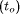and the maximum runtime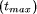of all subproblems are summarized for all CSPs of an individual model. The sums are presented in the corresponding rows of the table. All subproblems were solved with the simple backtracking algorithm as used in QSim.

A further interesting point is the influence of the number of generated subproblems toand. Three cases are considered --- the CSP is partitioned into at most 16, at most 64, and at most 256 subproblems. The corresponding runtimes are also presented in the tables.

Due to the exploitation of the dependencies between adjacent constraints, the VBP method achieves better results than CBP. Especially, the big increase of the overall runtimeand the size of the maximum subtasklead to poor parallel performance with CBP. VBP generates shorter maximum subtasks, and in some casesis shorter than the runtime of the single-processor algorithm.

Page 816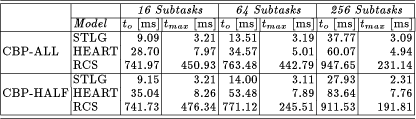Table 1: CBP method. All runtimes of CSPs of an individual QSim model are summarized and are presented in the corresponding row. The runtimes are measured on a digital signal processor TMS320C40. The runtimes for the single-processor algorithm are 6.12 ms for STLG, 25.72 ms for HEART, and 726 ms for RCS.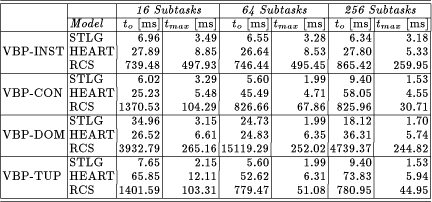Table 2: VBP method. All runtimes of CSPs of an individual QSim model are summarized and are presented in the corresponding row. The runtimes for the single-processor algorithm are 6.83 ms for STLG, 28.77 ms for HEART, and 805.63 ms for RCS. The small increase compared to the single-processor runtimes of CBP is due to different memory mappings of the target system.

Speedup Estimation of VBP

A comparison of the speedup estimation for VBP-INST and VBP-CON is shown in [Figure 2]. In most cases VBP-CON outperforms VBP-INST --- especially for complex CSPs (model RCS). VBP-CON results in a linear speedup for worst- and best-case estimation. It turns out that the length of the maximum task limits the expected speedup for VBP-INST.

Speedup increases with the number of generated tasks. However, the more tasks are generated the more overall communication time is required and the speedup of highly partitioned CSP can be lost. Best results are expected with VBP-CON and a medium number of tasks.

Page 817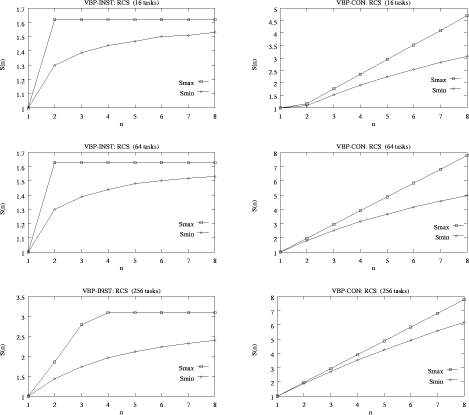Figure 2: Speedup estimation of VBP for the RCS model. Worst- and best-case speedup for VBP-INST and VBP-CON are shown in the left and right column plots. Especially for complex models VBP-CON performs better than VBP-INST.

4.3 Comparison of Single-Processor CSP Algorithms

The CSPs of the three QSim models have been solved with different sequential algorithms. We have tried to find a parameter to estimate the runtime of a given CSP. The average number of tuples per constraint (T/C) was chosen as such a parameter. A plot of the runtimes is presented in [Figure 3]. The CSPs are ordered corresponding to this parameter.

It turns out that simple backtracking is the fastest algorithm for simple QSim models. For complex models sophisticated algorithms perform better. Graphdirected backjumping (GBJ) has the shortest runtime on almost all complex models. Thus, the parameter T/C can be used to divide QSim CSPs into two parts. Simple CSPs (T/C is smaller than a given limit) should be solved with simple backtracking, the other CSPs should be solved with the GBJ algorithm.

Page 818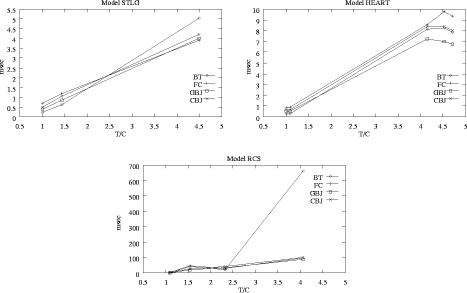Figure 3: Comparison of sequential backtracking algorithms. The CSPs are ordered to the average number of tuples per constraint (T/C). For simple CSPs BT performs better than the other algorithms. On more complex CSPs the opposite is true --- especially GBJ is up to 7 times faster than BT for RCS at T/C = 4.

5 Conclusions

In this paper we have presented a parallelizing strategy for constraint satisfaction in QSim. Two important steps of the PAB strategy are studied in detail. First, partitioning methods for the CSP are introduced and evaluated. The evaluation of these methods is based on runtime measurements of the subproblems and a worst- and best-case speedup estimation. Second, different sequential backtracking algorithms are compared using QSim CSPs. Results from this work can be summarized as follows.

VBP-CON partitioning method
VBP-CON performs better than the other partitioning methods. A medium number of generated subproblem should be chosen to achieve a good tradeoff between communication times and length of the maximum subtask.
BT and GBJ for solving the subproblems
Simple CSPs should be solved with simple backtracking, more complex CSP should be solved with graph-directed backjumping (GBJ). The complexity of a CSP can be estimated with the average number of tuples per constraint (T/C). The exact limit between BT and GBJ depends on the implementation of the algorithms and has to be determined experimentally.
Implementation of parallel constraint satisfaction based on the PAB strategy is in progress. The strategy is implemented on a multiprocessor system consisting of TMS320C40. The speedup estimations are compared with experimental results from this implementation.

Page 819

References

[Beck 94] Peter van Beck.: ``CSP Library''; Department of Computing Science, University of Alberta (1994), library of CSP algorithms.

[Conrad, Agrawal, Bahler 92] James M. Conrad, Dharma P. Agrawal, and Dennis R. Bahler.: ``Scalable Parallel Arc Consistency Algorithms for Shared Memory Computers''; Proceedings 6th International Parallel Processing Symposium, IEEE, Los Alamitos, CA, (1992), 242-249.

[Cooper, Swain 92] Paul R. Cooper and Michael J. Swain.: ``Arc consistency: parallelism and domain dependence''; Artificial Intelligence, 58 (1992), 207-235.

[Dechter 90] Rina Dechter.: ``Enhancement Schemes for Constraint Processing: Backjumping, Learning, and Cutset Decomposition''; Artificial Intelligence, 41 (1990), 273-312.

[Dechter 92] Rina Dechter.: ``Constraint Networks''; Stuart C. Shapiro, editor, Encyclopedia of Artificial Intelligence, John Wiley & Sons, 1 (1992), 276-285.

[Kay 92] Herbert Kay.: ``A qualitative model of the space shuttle reaction control system''; Technical Report AI92-188, Artificial Intelligence Laboratory, University of Texas (1992).

[Kondrak 94] Grzegorz Kondrak.: ``A Theoretical Evaluation of Selected Backtracking Algorithms''; Master's thesis, Department of Computing Science, University of Alberta (1994)

[Kuipers 94] Benjamin Kuipers.: ``Qualitative Reasoning: Modeling and Simulation with Incomplete Knowledge''; Artificial Intelligence, MIT Press (1994)

[Luo, Hendry, Buchanan 94] Q.P. Luo, P.G. Hendry, and J.T. Buchanan.: ``Strategies for Distributed Constraint Satisfaction Problems''; Proceedings 13th International DAI Workshop, DAI, Seattle, WA (1994) [Mackworth 77] Alan K. Mackworth.: ``Consistency in Networks of Relations''; Artificial Intelligence, 8 (1977), 99-118.

[Mohr, Henderson 86] Roger Mohr and Thomas C. Henderson.: ``Arc and Path Consistency Revised''; Artificial Intelligence, 28 (1986), 225-233.

[Platzner, Rinner, Weiss 95] Marco Platzner, Bernhard Rinner, and Reinhold Weiss.: ``A Distributed Computer Architecture for Qualitative Simulation Based on a Multi-DSP and FPGAs''; 3rd Euromicro Workshop on Parallel and Distributed Processing, IEEE Computer Society Press, San Remo (1995), 311-318.

[Prosser 93] Patrick Prosser.: ``Hybrid Algorithms for the Constraint Satisfaction Problem''; Computational Intelligence, 9, 3 (1993) 268-299.

[Rao, Kumar 93] V. Nageshwara Rao and Vipin Kumar.: ``On the Efficiency of Parallel Backtracking''; IEEE Transactions on Parallel and Distributed Systems, 4, 4(1993), 427-437.

[Riedl 95] Johannes Riedl.: ``Parallele Algorithmen und Laufzeitmessungen für Constraint Satisfaction im qualitativen Simulator QSim''; Master's thesis, Institute for Technical Informatics, Graz University of Technology (1995)

Acknowledgements

This project is partially supported by the Austrian National Science Foundation Fonds zur Förderung der wissenschaftlichen Forschung under grant number P10411-MAT.

Page 820